# Selina Solutions Concise Mathematics Class 6 Chapter 29: The Circle

Selina Solutions Concise Mathematics Class 6 Chapter 29 The Circle are readily available here. The subject matter experts at BYJU’S have outlined all the concepts of this chapter in a clear and precise manner to fulfill the needs of all students. The solutions provide numerous shortcut methods and tricks to explain all the exercise problems in an easily understandable language. Students also learn various techniques to solve all the exercise problems pertaining to the concepts using these solutions. Those who wish to secure high marks, are suggested to practice exercise problems with the help of solutions on a regular basis. For an effective understanding of the problems, students can find Selina Solutions Concise Mathematics Class 6 Chapter 29 The Circle PDF, from the links which are provided here.

Chapter 29 explains parts of a circle, concentric circle, chord of a circle, segment of a circle, arc of a circle and sector of a circle, with illustrations. By revising exercise problems using solutions, students can evaluate their hold on the concepts.

## Selina Solutions Concise Mathematics Class 6 Chapter 29 The Circle Download PDF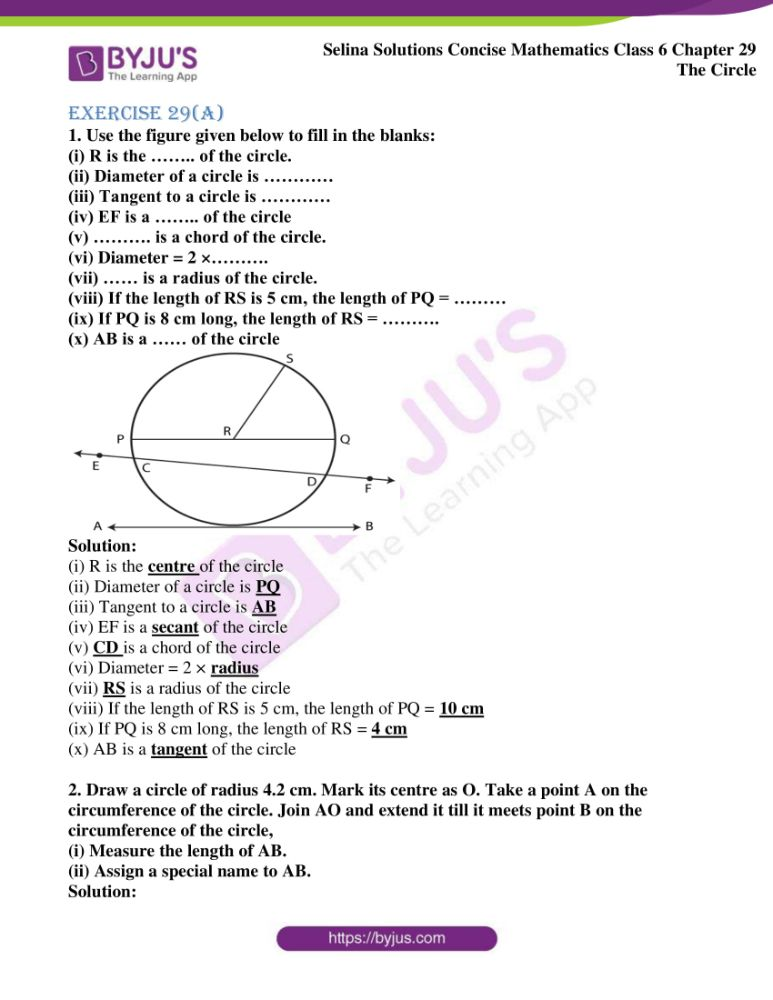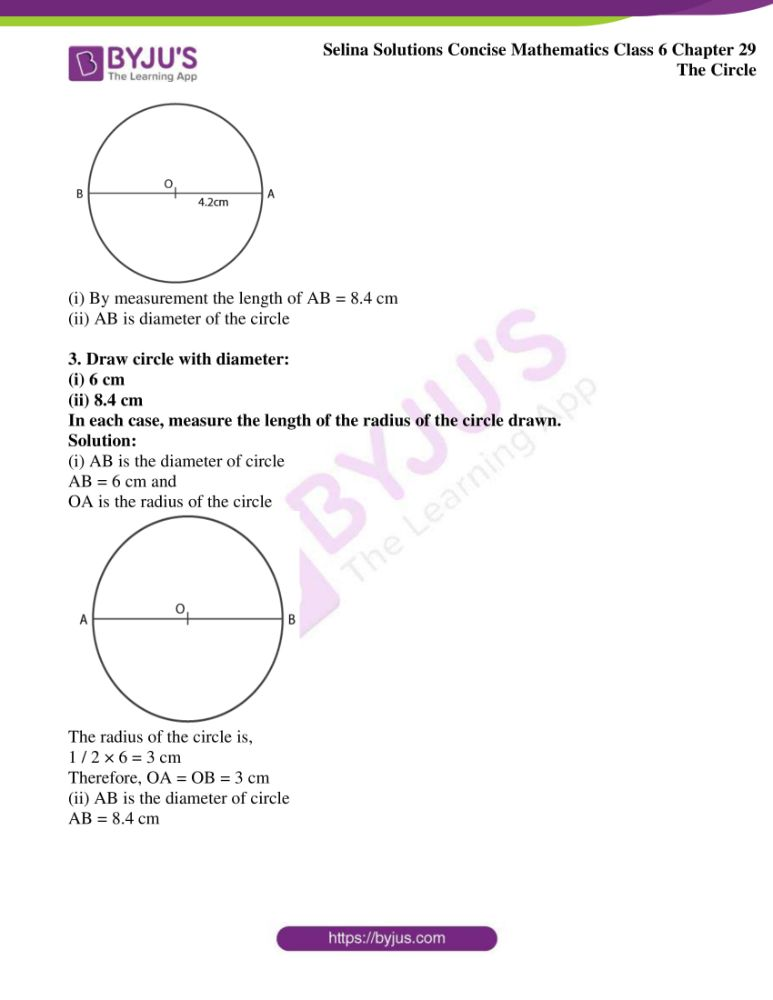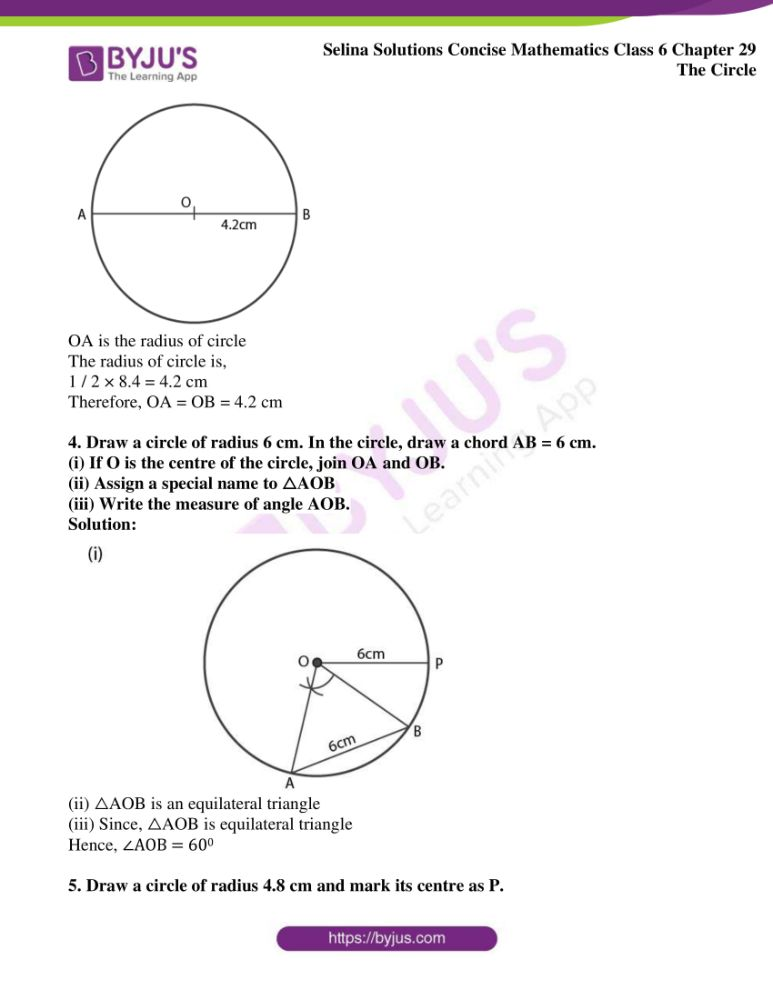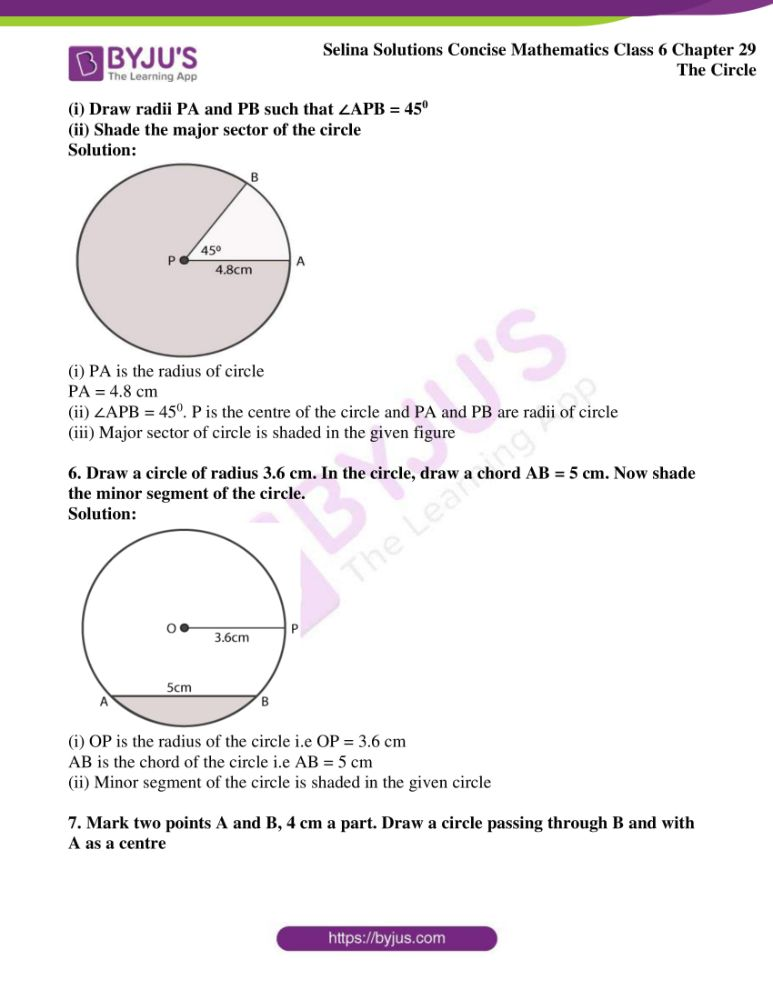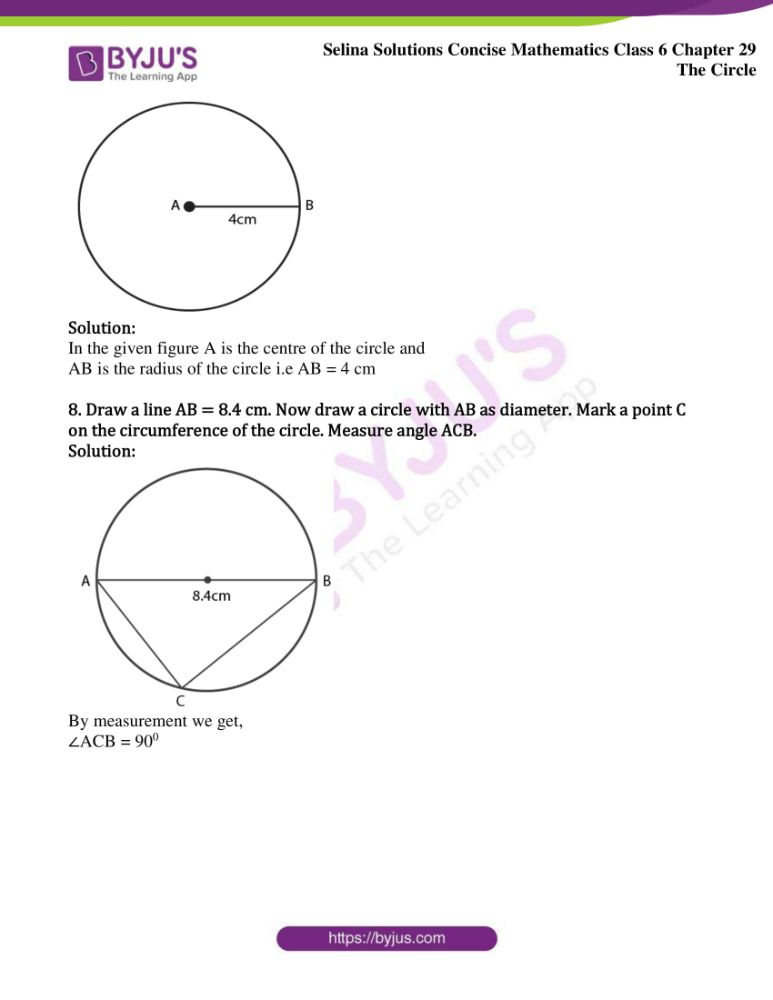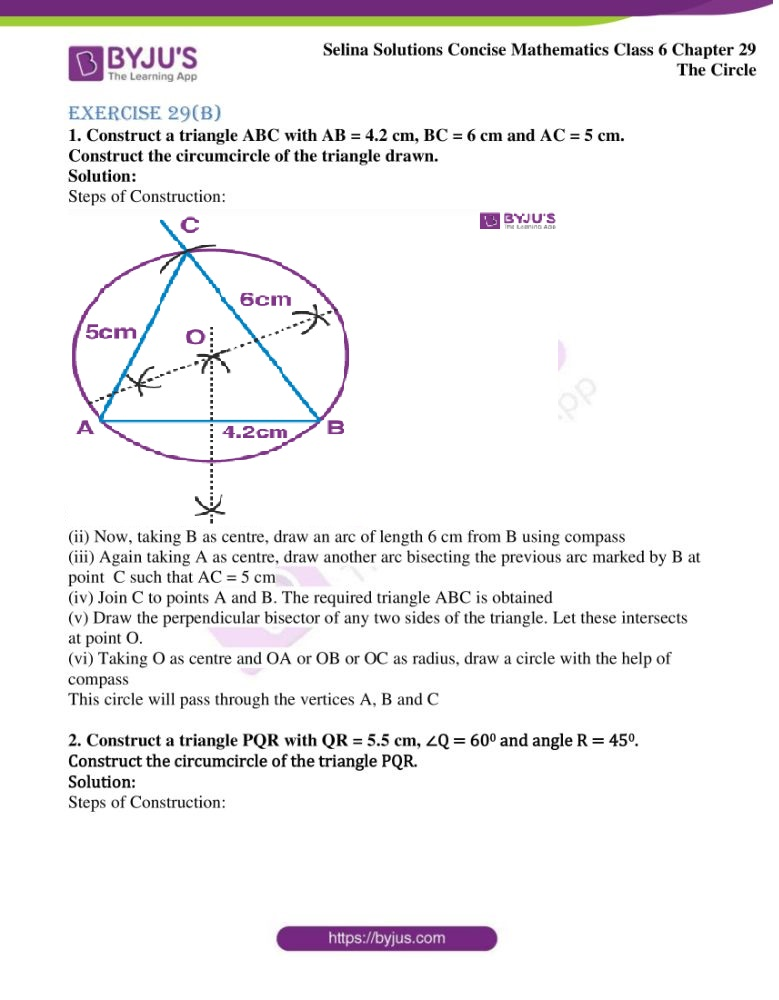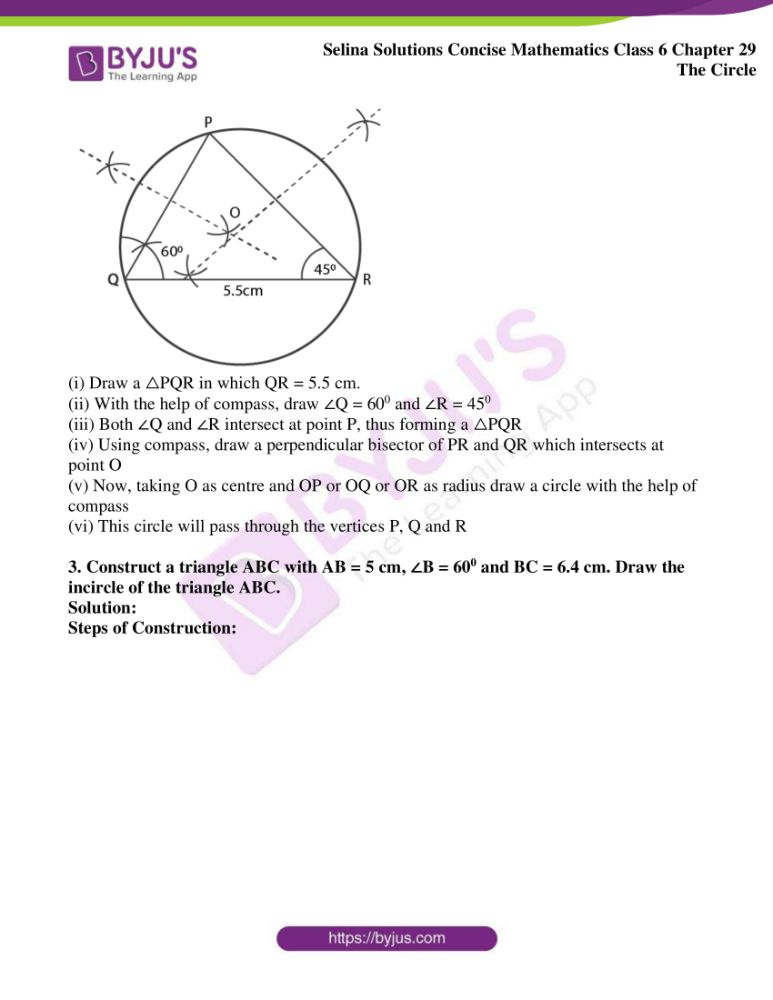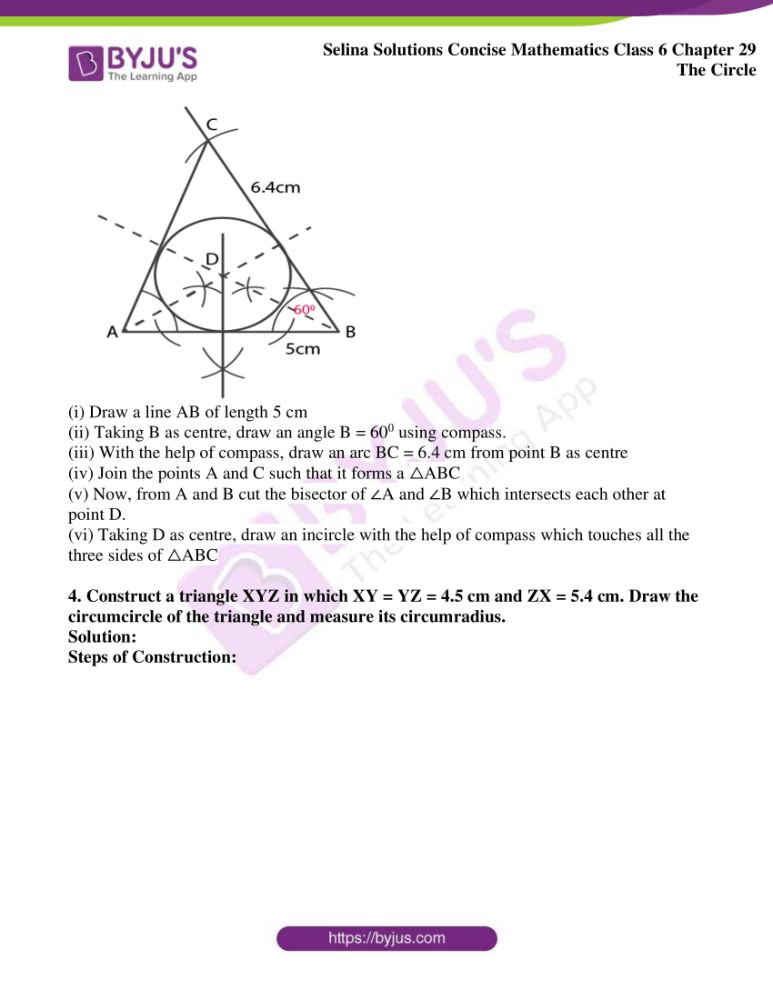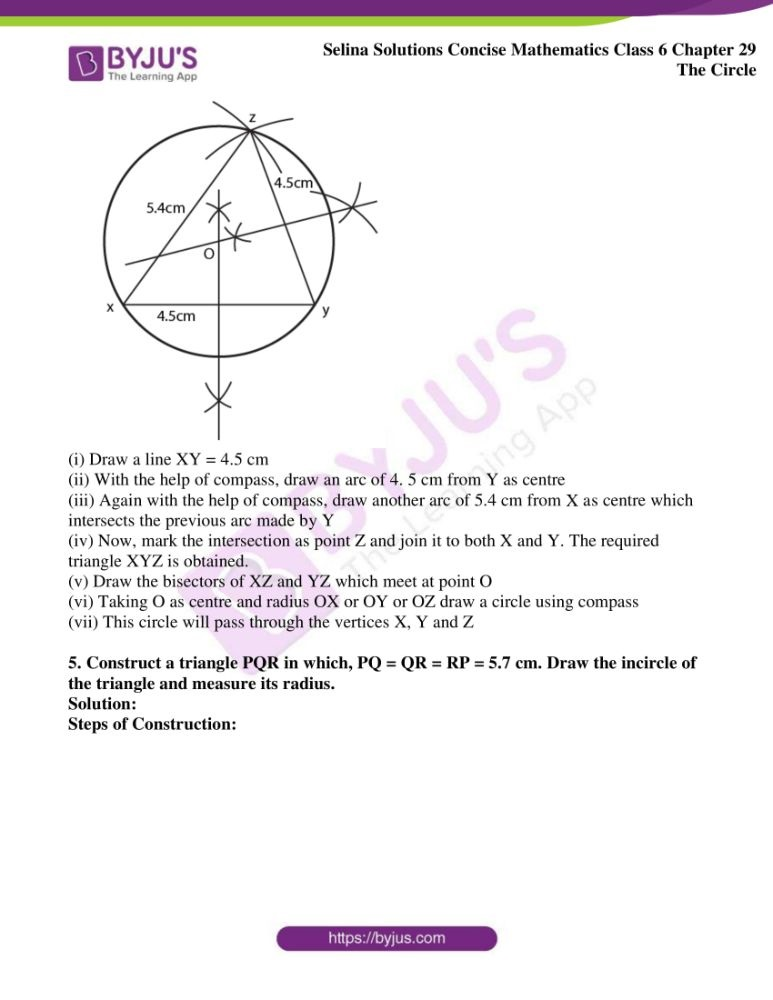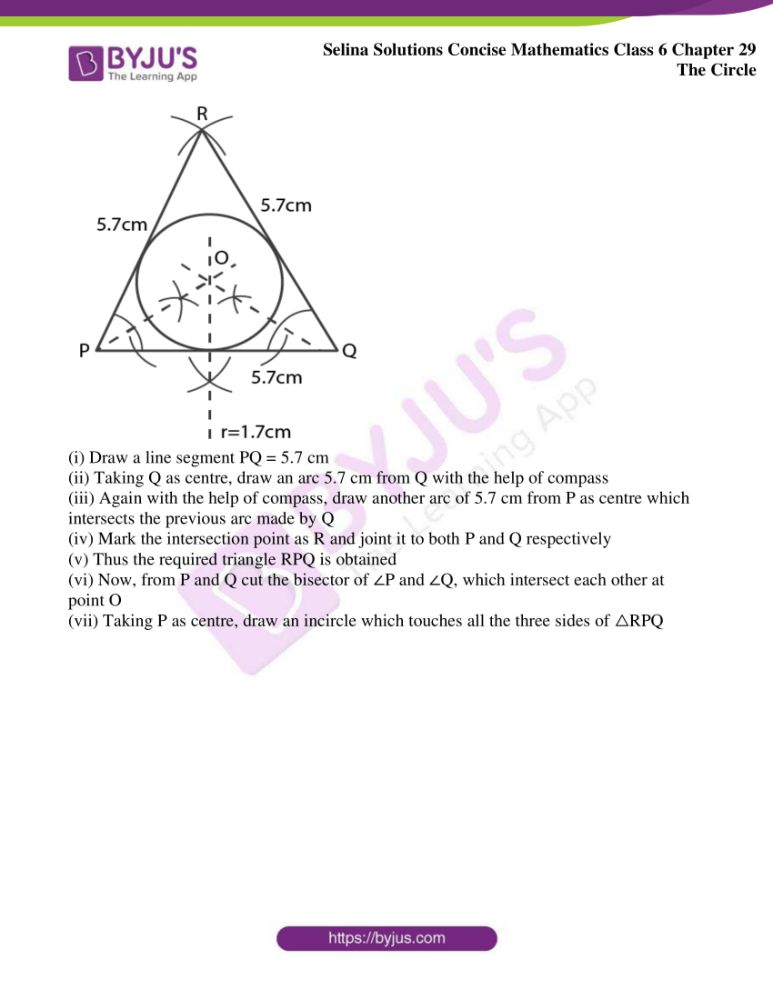### Exercises of Selina Solutions Concise Mathematics Class 6 Chapter 29 The Circle

Exercise 29(A) Solutions

Exercise 29(B) Solutions

## Access Selina Solutions Concise Mathematics Class 6 Chapter 29 The Circle

Exercise 29(A)

1. Use the figure given below to fill in the blanks:

(i) R is the …….. of the circle.

(ii) Diameter of a circle is …………

(iii) Tangent to a circle is …………

(iv) EF is a …….. of the circle

(v) ………. is a chord of the circle.

(vi) Diameter = 2 ×……….

(vii) …… is a radius of the circle.

(viii) If the length of RS is 5 cm, the length of PQ = ………

(ix) If PQ is 8 cm long, the length of RS = ……….

(x) AB is a …… of the circle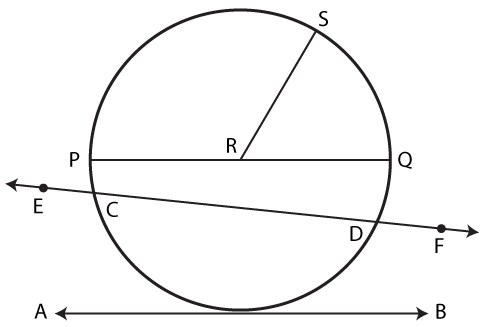Solution:

(i) R is the centre of the circle

(ii) Diameter of a circle is PQ

(iii) Tangent to a circle is AB

(iv) EF is a secant of the circle

(v) CD is a chord of the circle

(vi) Diameter = 2 × radius

(vii) RS is a radius of the circle

(viii) If the length of RS is 5 cm, the length of PQ = 10 cm

(ix) If PQ is 8 cm long, the length of RS = 4 cm

(x) AB is a tangent of the circle

2. Draw a circle of radius 4.2 cm. Mark its centre as O. Take a point A on the circumference of the circle. Join AO and extend it till it meets point B on the circumference of the circle,

(i) Measure the length of AB.

(ii) Assign a special name to AB.

Solution: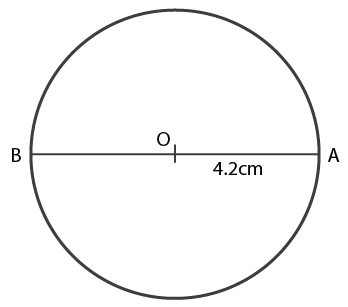(i) By measurement the length of AB = 8.4 cm

(ii) AB is diameter of the circle

3. Draw circle with diameter:

(i) 6 cm

(ii) 8.4 cm

In each case, measure the length of the radius of the circle drawn.

Solution:

(i) AB is the diameter of circle

AB = 6 cm and

OA is the radius of the circle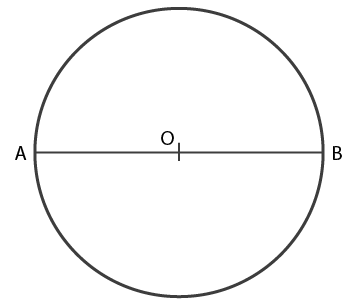The radius of the circle is,

1 / 2 × 6 = 3 cm

Therefore, OA = OB = 3 cm

(ii) AB is the diameter of circle

AB = 8.4 cm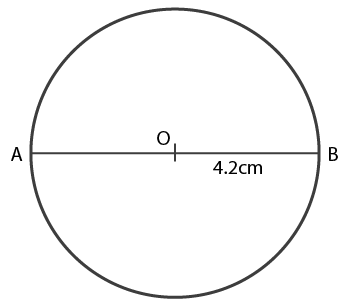OA is the radius of circle

1 / 2 × 8.4 = 4.2 cm

Therefore, OA = OB = 4.2 cm

4. Draw a circle of radius 6 cm. In the circle, draw a chord AB = 6 cm.

(i) If O is the centre of the circle, join OA and OB.

(ii) Assign a special name to △AOB

(iii) Write the measure of angle AOB.

Solution: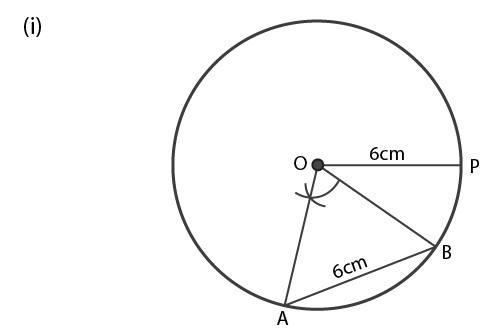(ii) △AOB is an equilateral triangle

(iii) Since, △AOB is equilateral triangle

Hence, ∠AOB = 600

5. Draw a circle of radius 4.8 cm and mark its centre as P.

(i) Draw radii PA and PB such that ∠APB = 450

(ii) Shade the major sector of the circle

Solution: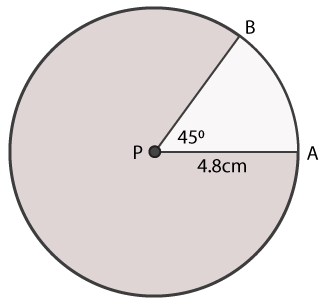(i) PA is the radius of circle

PA = 4.8 cm

(ii) ∠APB = 450. P is the centre of the circle and PA and PB are radii of circle

(iii) Major sector of circle is shaded in the given figure

6. Draw a circle of radius 3.6 cm. In the circle, draw a chord AB = 5 cm. Now shade the minor segment of the circle.

Solution: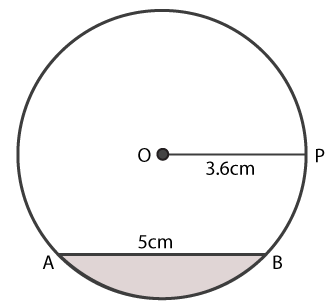(i) OP is the radius of the circle i.e OP = 3.6 cm

AB is the chord of the circle i.e AB = 5 cm

(ii) Minor segment of the circle is shaded in the given circle

7. Mark two points A and B, 4 cm a part. Draw a circle passing through B and with A as a centre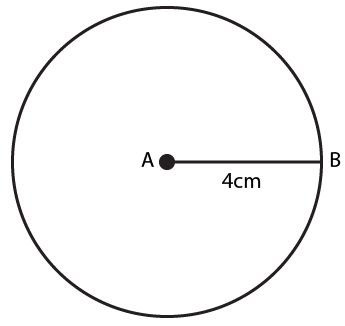Solution:

In the given figure A is the centre of the circle and

AB is the radius of the circle i.e AB = 4 cm

8. Draw a line AB = 8.4 cm. Now draw a circle with AB as diameter. Mark a point C on the circumference of the circle. Measure angle ACB.

Solution: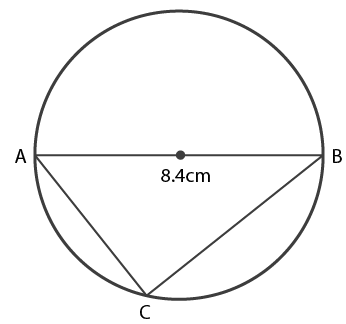By measurement we get,

∠ACB = 900

Exercise 29(B)

1. Construct a triangle ABC with AB = 4.2 cm, BC = 6 cm and AC = 5 cm. Construct the circumcircle of the triangle drawn.

Solution:

Steps of Construction: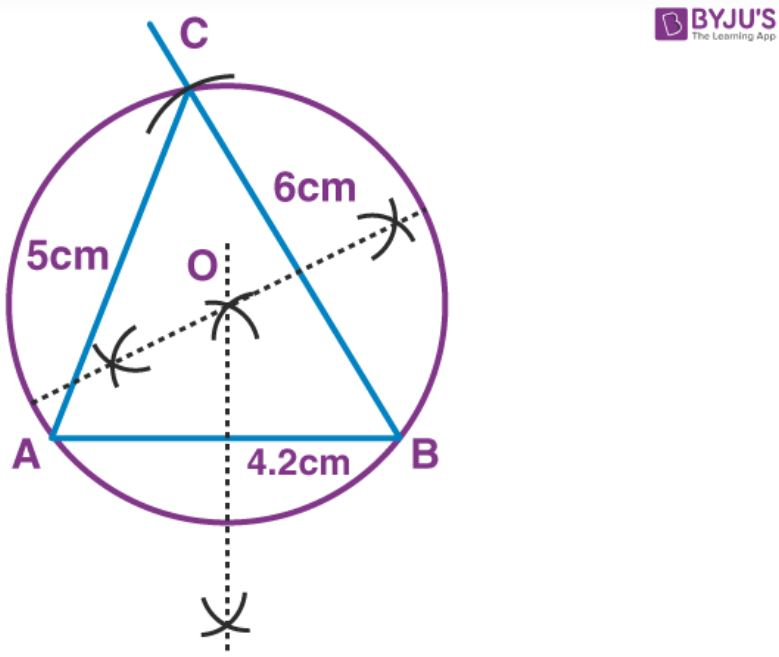(i) Draw a line segment AB = 4.2 cm

(ii) Now, taking B as centre, draw an arc of length 6 cm from B using compass

(iii) Again taking A as centre, draw another arc bisecting the previous arc marked by B at point C such that AC = 5 cm

(iv) Join C to points A and B. The required triangle ABC is obtained

(v) Draw the perpendicular bisector of any two sides of the triangle. Let these intersects at point O.

(vi) Taking O as centre and OA or OB or OC as radius, draw a circle with the help of compass

This circle will pass through the vertices A, B and C

2. Construct a triangle PQR with QR = 5.5 cm, ∠Q = 600 and angle R = 450. Construct the circumcircle of the triangle PQR.

Solution:

Steps of Construction: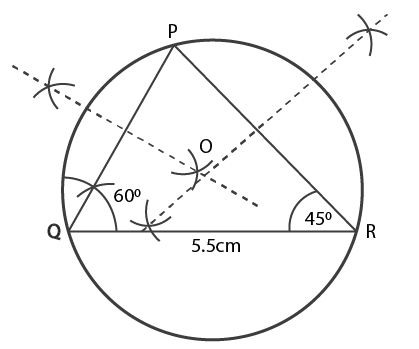(i) Draw a △PQR in which QR = 5.5 cm.

(ii) With the help of compass, draw ∠Q = 600 and ∠R = 450

(iii) Both ∠Q and ∠R intersect at point P, thus forming a △PQR

(iv) Using compass, draw a perpendicular bisector of PR and QR which intersects at point O

(v) Now, taking O as centre and OP or OQ or OR as radius draw a circle with the help of compass

(vi) This circle will pass through the vertices P, Q and R

3. Construct a triangle ABC with AB = 5 cm, ∠B = 600 and BC = 6.4 cm. Draw the incircle of the triangle ABC.

Solution:

Steps of Construction: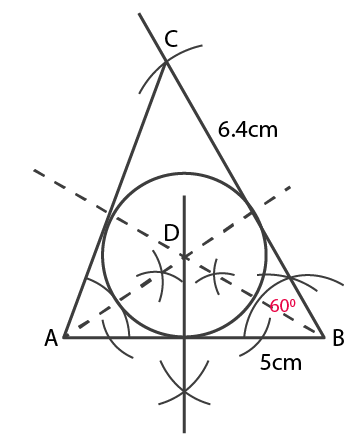(i) Draw a line AB of length 5 cm

(ii) Taking B as centre, draw an angle B = 600 using compass.

(iii) With the help of compass, draw an arc BC = 6.4 cm from point B as centre

(iv) Join the points A and C such that it forms a △ABC

(v) Now, from A and B cut the bisector of ∠A and ∠B which intersects each other at point D.

(vi) Taking D as centre, draw an incircle with the help of compass which touches all the three sides of △ABC

4. Construct a triangle XYZ in which XY = YZ = 4.5 cm and ZX = 5.4 cm. Draw the circumcircle of the triangle and measure its circumradius.

Solution:

Steps of Construction: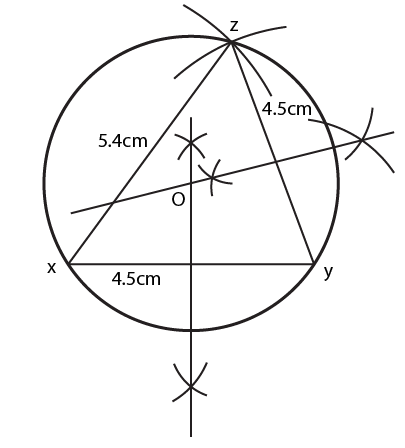(i) Draw a line XY = 4.5 cm

(ii) With the help of compass, draw an arc of 4. 5 cm from Y as centre

(iii) Again with the help of compass, draw another arc of 5.4 cm from X as centre which intersects the previous arc made by Y

(iv) Now, mark the intersection as point Z and join it to both X and Y. The required triangle XYZ is obtained.

(v) Draw the bisectors of XZ and YZ which meet at point O

(vi) Taking O as centre and radius OX or OY or OZ draw a circle using compass

(vii) This circle will pass through the vertices X, Y and Z

5. Construct a triangle PQR in which, PQ = QR = RP = 5.7 cm. Draw the incircle of the triangle and measure its radius.

Solution:

Steps of Construction: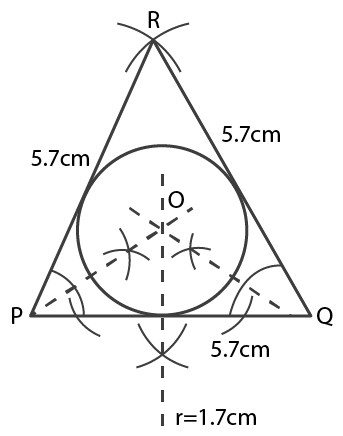(i) Draw a line segment PQ = 5.7 cm

(ii) Taking Q as centre, draw an arc 5.7 cm from Q with the help of compass

(iii) Again with the help of compass, draw another arc of 5.7 cm from P as centre which intersects the previous arc made by Q

(iv) Mark the intersection point as R and joint it to both P and Q respectively

(v) Thus the required triangle RPQ is obtained

(vi) Now, from P and Q cut the bisector of ∠P and ∠Q, which intersect each other at point O

(vii) Taking P as centre, draw an incircle which touches all the three sides of △RPQ